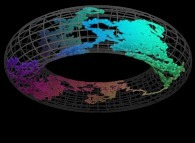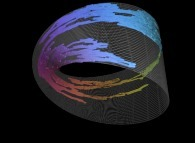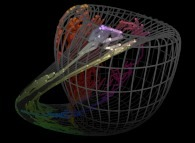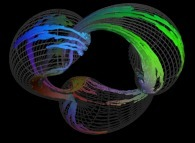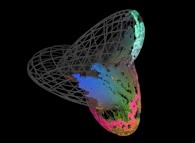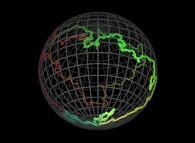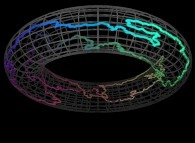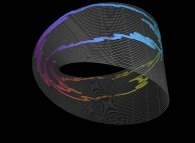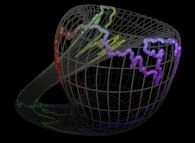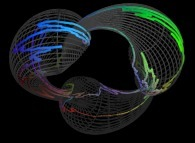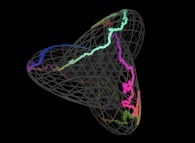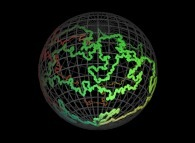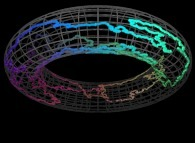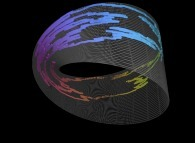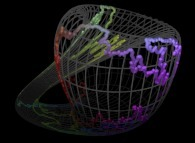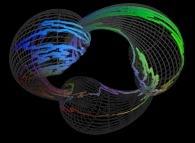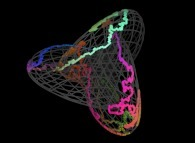# Hilbert and Peano Space filling Curves and Beyond: From Squares and Cubes to Surfaces (bidimensional Manifolds) and tridimensional Manifolds

## www.lactamme.polytechnique.fr

#### jean-francois.colonna@polytechnique.edu CMAP (Centre de Mathématiques APpliquées) UMR CNRS 7641, École polytechnique, Institut Polytechnique de Paris, CNRS, 91120 Palaiseau, France

[Site Map, Help and Search [Plan du Site, Aide et Recherche]]
[The Y2K Bug [Le bug de l'an 2000]]
[Do you believe that Real Numbers exist for a computer and that floating point computations are safe? [Croyez-vous que les Nombres Réels existent dans un ordinateur et que les calculs flottants sont sûrs?]]
[Please, visit A Virtual Machine for Exploring Space-Time and Beyond, the place where you can find thousands of pictures and animations between Art and Science]
(CMAP28 WWW site: this page was created on 01/03/2023 and last updated on 05/24/2023 18:16:54 -CEST-)

[en français/in french

Contents:

# 1-The bidimensional Peano Surjection:

Giuseppe Peano defined the following surjection :
```                    [0,1] --> [0,1]x[0,1]
```
Let's T being a real number defined using the base 3 :
```                    T    = 0.A1A2A3... ∈ [0,1] with Ai ∈ {0,1,2}
```
Let's X(T) and Y(T) being two real functions of T defined as :
```                    X(T) = 0.B1B2B3... ∈ [0,1] with Bi ∈ {0,1,2}
```
```                    Y(T) = 0.C1C2C3... ∈ [0,1] with Ci ∈ {0,1,2}
```
with :
```                    Bn = A2n-1 if A2+A4+...+A2n-0 is even
```
```                    Bn = 2-A2n-1 otherwise
```

```                    Cn = A2n if A1+A3+...+A2n-1 is even
```
```                    Cn = 2-A2n otherwise
```

These two functions X(T) and Y(T) are the coordinates of a point P(T) inside the [0,1]x[0,1] square. The displayed "curve" -as little spheres- is the trajectory of P(T) when T varies from 0 (lower left corner) to 1-epsilon (upper right corner).

Here are the four first bidimensional Peano curves with an increasing number of digits {2,4,6,8}: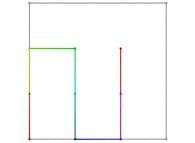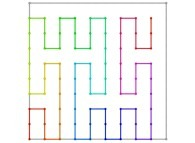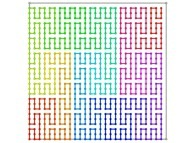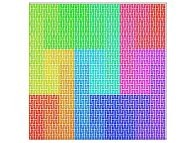[See the used color set to display the parameter T]

# 2-The tridimensional Peano Surjection:

A tridimensional surjection can be defined :
```                    [0,1] --> [0,1]x[0,1]x[0,1]
```
as a generalization of the bidimensional one.

Let's T being a real number defined using the base 3 :
```                    T    = 0.A1A2A3... ∈ [0,1] with Ai ∈ {0,1,2}
```
Let's X(T), Y(T) and Z(T) being three real functions of T defined as:
```                    X(T) = 0.B1B2B3... ∈ [0,1] with Bi ∈ {0,1,2}
```
```                    Y(T) = 0.C1C2C3... ∈ [0,1] with Ci ∈ {0,1,2}
```
```                    Y(T) = 0.D1D2D3... ∈ [0,1] with Di ∈ {0,1,2}
```
with :
```                    Bn = A3n-2 if A3+A6+...+A3n-0 is even
```
```                    Bn = 2-A3n-2 otherwise
```

```                    Cn = A3n-1 if A2+A5+...+A3n-1 is even
```
```                    Cn = 2-A3n-1 otherwise
```

```                    Dn = A3n if A1+A4+...+A3n-2 is even
```
```                    Dn = 2-A3n otherwise
```

These three functions X(T), Y(T) and Z(T) are the coordinates of a point P(T) inside the [0,1]x[0,1]x[0,1] cube. The displayed "curve" is the trajectory of P(T) -displayed as little spheres- when T varies from 0 (lower left corner) to 1-epsilon (upper right corner).

Here are the three first tridimensional Peano curves with an increasing number of digits {3,6,9}: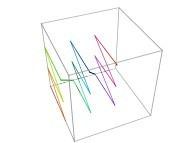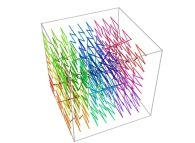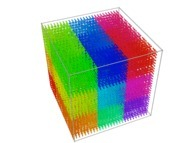[See the used color set to display the parameter T]

# 3-The bidimensional Hilbert Curves:

Let's C1(T) being a parametric curve defined by means of 2 real functions of T (T [0,1]) X1(T) [0,1] and Y1(T) [0,1 such as :
```                    X1(T=0)=0 Y1(T=0)=0 (lower left corner)
```
```                    X1(T=1)=1 Y1(T=1)=0 (lower right corner)
```

Then one defines a sequence of curves Ci(T) (i >= 1) as follows :
```                    Ci(T) = {Xi(T),Yi(T)} ∈ [0,1]x[0,1] --> Ci+1(T) = {Xi+1(T),Yi+1(T)} ∈ [0,1]x[0,1]
```

```                    if T ∈ [0,1/4[:
Xi+1(T) =   Yi(4T-0)
Yi+1(T) =   Xi(4T-0)
Transformation 1
```
```                    if T ∈ [1/4,2/4[:
Xi+1(T) =   Xi(4T-1)
Yi+1(T) = 1+Yi(4T-1)
Transformation 2
```
```                    if T ∈ [2/4,3/4[:
Xi+1(T) = 1+Xi(4T-2)
Yi+1(T) = 1+Yi(4T-2)
Transformation 3
```
```                    if T ∈ [3/4,1]:
Xi+1(T) = 2-Yi(4T-3)
Yi+1(T) = 1-Xi(4T-3)
Transformation 4
```

Please note that 4=2d where d=2 is the space dimension.

See a special C1(T) curve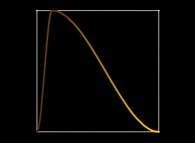in order to understand the geometrical meaning of the 4 transformations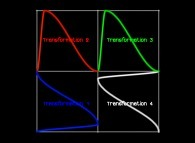and of their order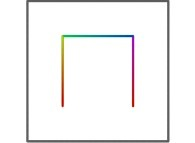.

Here are the five first bidimensional Hilbert curves with an increasing number of iterations :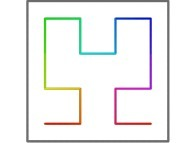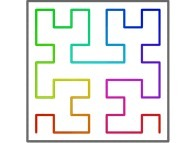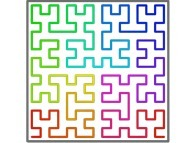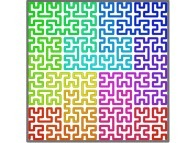[See the used color set to display the parameter T]

Here are some examples of Hilbert-like bidimensional curves :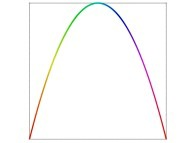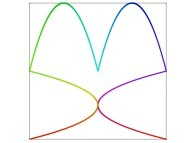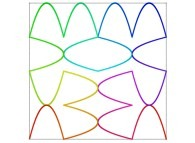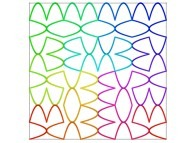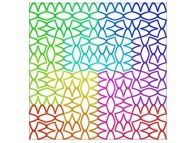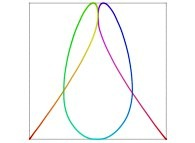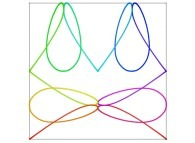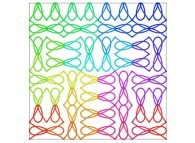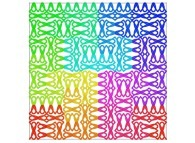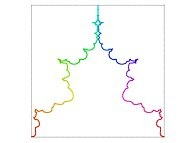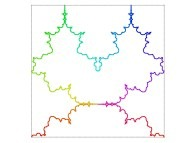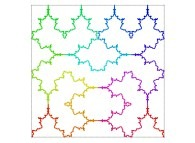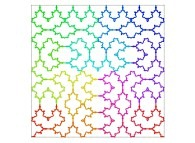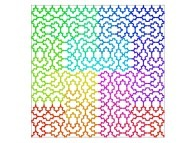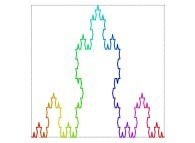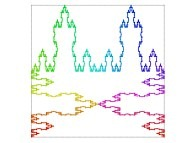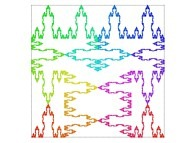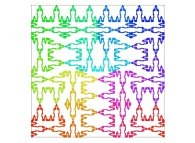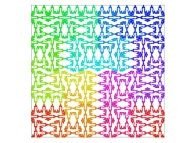# 4-The tridimensional Hilbert Curves:

Let's C1(T) being a parametric curve defined by means of 3 real functions of T (T [0,1]) X1(T) [0,1], Y1(T) [0,1] and Z1(T) [0,1 such as :
```                    X1(T=0)=0 Y1(T=0)=0 Z1(T=0)=0 (lower left foreground corner)
```
```                    X1(T=1)=0 Y1(T=1)=0 Z1(T=1)=1 (lower right foreground corner)
```

Then one defines a sequence of curves Ci(T) (i >= 1) as follows :
```                    Ci(T) = {Xi(T),Yi(T),Zi(T)} ∈ [0,1]x[0,1]x[0,1] --> Ci+1(T) = {Xi+1(T),Yi+1(T),Zi+1(T)} ∈ [0,1]x[0,1]x[0,1]
```

```                    if T ∈ [0,1/8[:
Xi+1(T) =   Xi(8T-0)
Yi+1(T) =   Zi(8T-0)
Zi+1(T) =   Yi(8T-0)
Transformation 1
```
```                    if T ∈ [1/8,2/8[:
Xi+1(T) =   Zi(8T-1)
Yi+1(T) = 1+Yi(8T-1)
Zi+1(T) =   Xi(8T-1)
Transformation 2
```
```                    if T ∈ [2/8,3/8[:
Xi+1(T) = 1+Xi(8T-2)
Yi+1(T) = 1+Yi(8T-2)
Zi+1(T) =   Zi(8T-2)
Transformation 3
```
```                    if T ∈ [3/8,4/8[:
Xi+1(T) = 1+Zi(8T-3)
Yi+1(T) = 1-Xi(8T-3)
Zi+1(T) = 1-Yi(8T-3)
Transformation 4
```
```                    if T ∈ [4/8,5/8[:
Xi+1(T) = 2-Zi(8T-4)
Yi+1(T) = 1-Xi(8T-4)
Zi+1(T) = 1+Yi(8T-4)
Transformation 5
```
```                    if T ∈ [5/8,6/8[:
Xi+1(T) = 1+Xi(8T-5)
Yi+1(T) = 1+Yi(8T-5)
Zi+1(T) = 1+Zi(8T-5)
Transformation 6
```
```                    if T ∈ [6/8,7/8[:
Xi+1(T) = 1-Zi(8T-6)
Yi+1(T) = 1+Yi(8T-6)
Zi+1(T) = 2-Xi(8T-6)
Transformation 7
```
```                    if T ∈ [7/8,1]:
Xi+1(T) =   Xi(8T-7)
Yi+1(T) = 1-Zi(8T-7)
Zi+1(T) = 2-Yi(8T-7)
Transformation 8
```

Please note that 8=2d where d=3 is the space dimension.

See a special C1(T) curve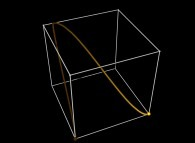in order to understand the geometrical meaning of the 8 transformations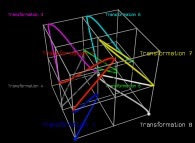and of their order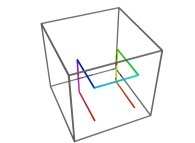.

Here are the four first tridimensional Hilbert curves with an increasing number of iterations :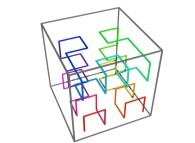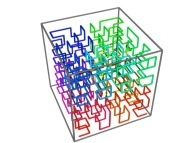[See the used color set to display the parameter T]

Here are some examples of Hilbert-like tridimensional curves :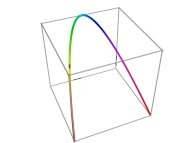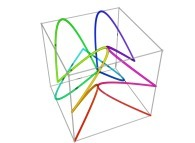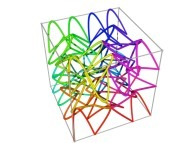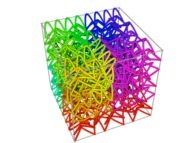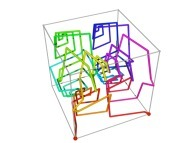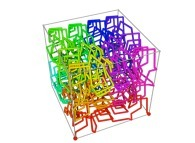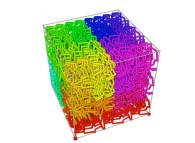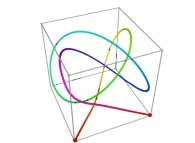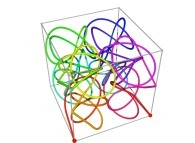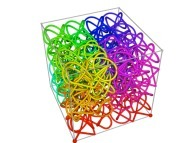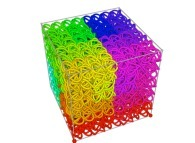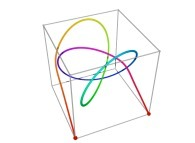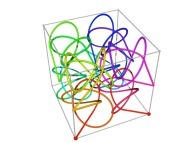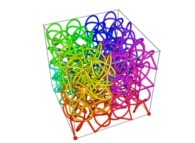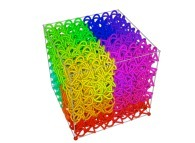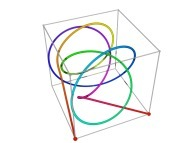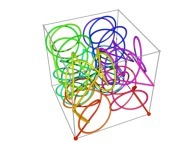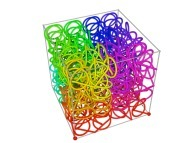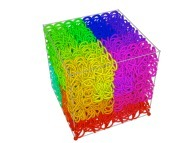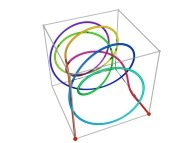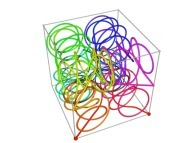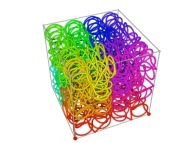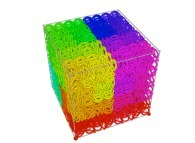[Plus d'informations à propos des Courbes de Peano et des Nœuds Infinis -en français/in french-]

# 5-Tridimensional Surfaces (Bidimensional Manifolds):

Many surfaces -bidimensional manifolds- in a tridimensional space can be defined using a set of three equations:
```                    X = Fx(u,v)
```
```                    Y = Fy(u,v)
```
```                    Z = Fz(u,v)
```
with:
```                    u ∈ [Umin,Umax]
```
```                    v ∈ [Vmin,Vmax]
```
For example:
```                    Fx(u,v) = R.sin(u).cos(v)
```
```                    Fy(u,v) = R.sin(u).sin(v)
```
```                    Fz(u,v) = R.cos(u)
```
with:
```                     u ∈ [0,pi]
```
```                     v ∈ [0,2.pi]
```
defines a sphere with R as the radius and the origin of the coordinates as the center.

[Umin,Umax]*[Vmin,Vmax] then defined a bidimensional rectangular domain D.
```                       v ^
|
V    |...... ---------------------------
max |      |+++++++++++++++++++++++++++|
|      |+++++++++++++++++++++++++++|
|      |+++++++++++++++++++++++++++|
|      |+++++++++++++++++++++++++++|
|      |+++++++++++++++++++++++++++|
|      |+++++++++++++++++++++++++++|
|      |+++++++++++++++++++++++++++|
|      |+++++++++++++++++++++++++++|
|      |+++++++++++++++++++++++++++|
V    |...... ---------------------------
min |      :                           :
|      :                           :
O------------------------------------------------->
U                           U              u
min                         max
```

Let's define a curve that fills the [0,1]x[0,1] square :
```                         ^
|
1 |---------------
| +++++   +++++ |
| +   +   +   + |
| +   +++++   + |
| +           + |
| +++++   +++++ |
|     +   +     |
| +++++   +++++ |
0 O------------------------>
0               1
```

Obviously one can define a mapping between the [0,1]x[0,1] square and the [Umin,Umax]*[Vmin,Vmax] domain :
```                       v ^
|
V    |...... ---------------------------
max |      | ++++++++         ++++++++ |
|      | +      +         +      + |
|      | +      +         +      + |
|      | +      +++++++++++      + |
|      | +           C           + |
|      | ++++++++         ++++++++ |
|      |        +         +        |
|      |        +         +        |
|      | ++++++++         ++++++++ |
V    |...... ---------------------------
min |      :                           :
|      :                           :
O------------------------------------------------->
U                           U              u
min                         max
```
Then it suffices to display only the points {Fx(u,v),Fy(u,v),Fz(u,v)} with {u,v} on the preceding curve C to fill the surface with C....

Here are some examples of this process :

 Surface ==> C Curve ==> Surface filling Curve

# 6-Tridimensional Manifolds:

Many tridimensional manifolds in a tridimensional space can be defined using a set of three equations:
```                    X = Fx(u,v,w)
```
```                    Y = Fy(u,v,w)
```
```                    Z = Fz(u,v,w)
```
with:
```                    u ∈ [Umin,Umax]
```
```                    v ∈ [Vmin,Vmax]
```
```                    w ∈ [Wmin,Wmax]
```
[Umin,Umax]*[Vmin,Vmax]*[Wmin,Wmax] then defined a tridimensional rectangular domain D.

It is obvious to generalize the preceding bidimensional process in the tridimensional space...

Here are some examples of this process :

 Tridimensional Manifold ==> C Curve ==> Tridimensional Manifold filling Curve

# 7-Beyond:

Instead of using space-filling curves in order to fill the {u,v} and {u,v,w} bi- and tridimensional domains, obviously one can use any means available and, for example, the bi- and the tridimensional brownian motions respectively....

Here are some examples of these extended processes :